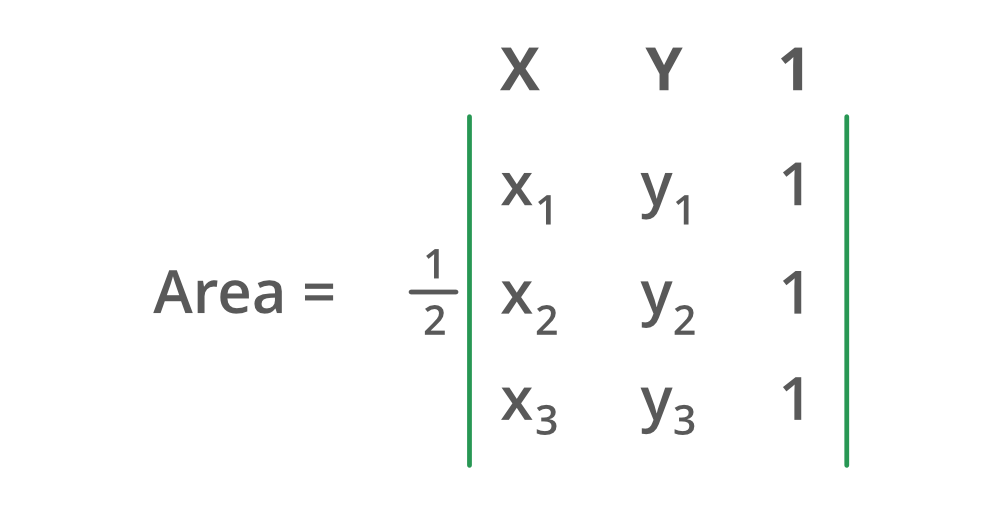# Java Program to Compute the Area of a Triangle Using Determinants

In order to compute the area of the triangle, two parameters are needed namely base and height. For this simply the variables are assigned with zeros.  The area of the triangle can be computed by using the same third-grade mathematics formula.

`Area = 1/2(Base * Height)`

Illustration:

Input: (1,3) (5,-2) (-3,6)

Output: Area of triangle: 4

Processing: 1/2 (|3*3 Determinant|)

Determinant: In linear algebra, the determinant is a scalar value that can be computed from the elements of a square matrix and encodes certain properties of the linear transformation described by the matrix. Matrix is simply a twodimensional array. Arrays are linear data structures in which elements are stored in a contiguous manner.

Algorithm:

1. Co-ordinates are asked from the user one by one
2. 1 is assigned to the third column in the matrix
3. Math library is imported for computation of numbers using formula over input elements
4. The absolute method is applied over the value stored in a variableSyntax:

`datatype typename = Math.abs(value)`

Return type: A value on the number line lying on the right side of 0

Math is a library in java for computing calculations over numbers which consist of function absolute whose use is to covert value being positive or negative to a positive value. Here it is used as a practically thinking area that can never be negative in real space. If something is occupying space it will definitely occupy space and will have the area.

Implementation: Java program to compute the area of a triangle

## Java

 `// java program for computing area of` `// triangle by using determinant`   `// Importing generic libraries` `import` `java.io.*;` `import` `java.util.*;`   `class` `GFG {`   `    ``// Function to compute area of triangle` `    ``static` `double` `area(``double``[][] a)` `    ``{`   `        ``// Formula for calculating triangle area` `        ``// using math library absolute function` `        ``double` `area = (Math.abs((a[``0``][``0``] * a[``1``][``1``])` `                                ``- (a[``0``][``0``] * a[``2``][``1``])` `                                ``+ (a[``1``][``0``] * a[``2``][``1``])` `                                ``- (a[``1``][``0``] * a[``0``][``1``])` `                                ``+ (a[``2``][``0``] * a[``0``][``1``])` `                                ``- (a[``2``][``0``] * a[``1``][``1``])))` `                      ``/ ``2``;`   `        ``// Return area of triangle` `        ``return` `area;` `    ``}`   `    ``// Main driver method` `    ``public` `static` `void` `main(String[] args)` `    ``{`   `        ``// Scanner for taking input from user` `        ``Scanner scan = ``new` `Scanner(System.in);`   `        ``// hard coded Input to show working`   `        ``// Storing vertices in a 3 * 3 matrix` `        ``// store x and y coordinate first then 1` `        ``double``[][] vertices` `            ``= { { ``1``, ``1``, ``1` `}, { ``1``, ``3``, ``1` `}, { ``3``, ``1``, ``1` `} };` `        ``// example x1=3 and y1 =1, then` `        ``// store them like {3, 1, 1}`   `        ``// Calling area function by passing vertices as` `        ``// parameters and storing in big data-type` `        ``double` `Area = area(vertices);`   `        ``// Print area of triangle on screen` `        ``System.out.println(``"Area of triangle: "` `+ Area);` `    ``}` `}`

Output

`Area of triangle: 2.0`

Time Complexity: O(1) since performing constant operations

Auxiliary Space: O(1)

Feeling lost in the vast world of Backend Development? It's time for a change! Join our Java Backend Development - Live Course and embark on an exciting journey to master backend development efficiently and on schedule.
What We Offer:
• Comprehensive Course
• Expert Guidance for Efficient Learning
• Hands-on Experience with Real-world Projects
• Proven Track Record with 100,000+ Successful Geeks

Previous
Next